Chapter Notes - Comparing Quantities

# Chapter Notes - Comparing Quantities Notes | Study Mathematics (Maths) Class 7 - Class 7

## Document Description: Chapter Notes - Comparing Quantities for Class 7 2022 is part of Mathematics (Maths) Class 7 preparation. The notes and questions for Chapter Notes - Comparing Quantities have been prepared according to the Class 7 exam syllabus. Information about Chapter Notes - Comparing Quantities covers topics like and Chapter Notes - Comparing Quantities Example, for Class 7 2022 Exam. Find important definitions, questions, notes, meanings, examples, exercises and tests below for Chapter Notes - Comparing Quantities.

Introduction of Chapter Notes - Comparing Quantities in English is available as part of our Mathematics (Maths) Class 7 for Class 7 & Chapter Notes - Comparing Quantities in Hindi for Mathematics (Maths) Class 7 course. Download more important topics related with notes, lectures and mock test series for Class 7 Exam by signing up for free. Class 7: Chapter Notes - Comparing Quantities Notes | Study Mathematics (Maths) Class 7 - Class 7
 1 Crore+ students have signed up on EduRev. Have you?

8. Comparing Quantities

Ratios and Proportions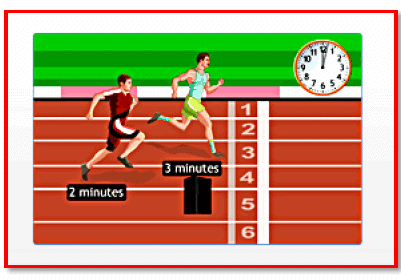Ratios are used to compare quantities.

Ratios are used to compare quantities. Ratios help us to compare quantities and determine the relation between them. We write ratios in the form of fractions, and then compare them by converting them into like fractions. If these like fractions are equal, then we say that the given ratios are equivalent.

Eg:6 pens cost Rs 90. What would be the cost 10 such pens?

Solution: Cost of 6 pens :Rs 90 Cost of 1 pen = 90/6=Rs 15 Hence, cost of 10 pens =10×15=150

The ratio of two quantities in the same unit is a fraction that shows how many times one quantity is greater or smaller than the other. When two ratios are equivalent, the four quantities are said to be in proportion.

Ratio and proportion problems can be solved by using two methods, the unitary method and equating the ratios to make proportions, and then solving the equation.

Percentages:

Percentage is another method used to compare quantities. Percentages are numerators of fractions with the denominator 100.

Page 31

Meaning of percentage:

fromPer cent is derived the Latin word 'per centum', which means per hundred. Per cent is represented by the symbol - %.

Percentages

Percentage is the numerator of a fraction, whose denominator is hundred.

Converting fractional numbers into percentage:

To compare fractional numbers, we need a common denominator. To convert a fraction into a percentage, multiply it by hundred and then place the % symbol.

For example, we have fraction 4/5

Percentages related to proper fractions are less than 100, whereas percentages related to improper fractions are more than 100.

Converting decimals into percentage:

To convert a decimal into percentage, multiply the decimal by 100%.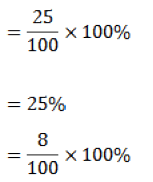Conversion of percentages into fractions or decimals:

Examples:

 Percent 2% 45% Fraction 2/100 45/100 Decimal 0.02 0.45

Conversions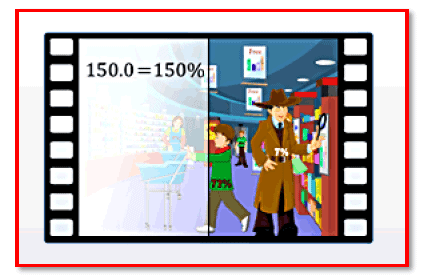A given percentage can be converted into fractions and decimals. Also, a decimal can be converted as percentage. Let us see one by one.

A given percentage can be converted into fractions and decimals. Also, a decimal can be converted as percentage. Let us see one by one.

To convert a percentage into a fraction:

Step1: Drop the percent sign, and then divide the number by hundred.

To convert a percentage into a decimal:

Step 1: Remove the percent sign.

Step 2: Divide the number by 100, or move the decimal point two places to the left in the numerator.

Eg: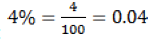To convert a decimal into a percentage:

Step1: Convert the decimal into a fraction.

Step 2: Multiply the fraction by hundred.

Step 3: Put a percent sign next to the number. Else, shift the decimal point two places to the right.

Page 33

Application of PercentagesPercentages are helpful in comparison.

Percentages are helpful in comparison. To calculate percentage increase/decrease, we use the formula:

Percentage increase/decrease = Profit = Selling price -Cost price ; Loss = Cost price-Selling price

Profit percentage or loss percentage is always calculated on the cost price.

1. To find the profit percent, we use the following formula: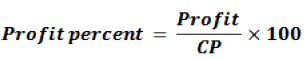2. To find the loss percent, we use the following formula:

Amount at the end of the year can be obtained by adding the sum borrowed (principal) and the interest. Thus, Amount = Principal + Interest The way of calculating interest, where the principal is not changed, is known as "simple interest".

As the number of years increases, interest will also increase. If P denotes the principal, R denotes the rate of interest and T denotes the time period, then the simple interest I paid for T years is given by If you are given any three of these quantities, the fourth one can be calculated using this formula.

The document Chapter Notes - Comparing Quantities Notes | Study Mathematics (Maths) Class 7 - Class 7 is a part of the Class 7 Course Mathematics (Maths) Class 7.
All you need of Class 7 at this link: Class 7

## Mathematics (Maths) Class 7

168 videos|252 docs|45 tests
 Use Code STAYHOME200 and get INR 200 additional OFF

## Mathematics (Maths) Class 7

168 videos|252 docs|45 tests

Track your progress, build streaks, highlight & save important lessons and more!

,

,

,

,

,

,

,

,

,

,

,

,

,

,

,

,

,

,

,

,

,

;